搜索内容 搜索用户

# [我分享] 相关向量机 (Relevance Vector Machine, RVM)[复制链接]iqiukp 发表于 2018-11-12 08:19:42
 写在开头：本帖会持续回帖更新，详情请看置顶2楼。 相关向量机 (Relevance Vector Machine, RVM) 在回归预测中的应用。 主要功能有： （1）基于SB2_Release_200工具箱 （2）输出训练数据和测试数据的预测值 （3）输出相关向量的序号和对应的数值 （4）输出预测值的均值和方差（即分布） 核心函数： （1）rvm_train function [model]= rvm_train(X,Y) % DESCRIPTION % Prediction based on Relevance Vector Machine (RVM) % Using SB2_Release_200 toolbox % % [model]= rvm_train(X,Y) % % INPUT % X Training samples (N*d) % N: number of samples % d: number of features % Y Target samples (N*1) % % OUTPUT % model RVM model % % % kernei width sigma = 5.5; L = size(X,1); % BASIS = [ones(L,1),computeKM(X,X,sigma)]; % % SETTINGS = SB2_ParameterSettings('NoiseStd',0.1); OPTIONS = SB2_UserOptions('diagnosticLevel','medium','monitor',10, ... 'diagnosticFile', 'logfile.txt'); [PARAMETER, HYPERPARAMETER, DIAGNOSTIC] = ... SparseBayes('Gaussian', BASIS, Y, OPTIONS); model.rv_index = PARAMETER.Relevant; model.rv_mu = PARAMETER.Value; model.width = sigma; model.X = X; model.beta = HYPERPARAMETER.beta; model.sigma = DIAGNOSTIC.Sigma; % mean of prediction (training samples) model.y_mu = BASIS(:,model.rv_index)*model.rv_mu; % variance of prediction (training samples) model.y_var = ones(L,1)*model.beta^-1+ ... diag(BASIS(:,model.rv_index)* ... model.sigma*BASIS(:,model.rv_index)'); end复制代码（2）rvm_test function [y_mu,y_var] = rvm_test(model,X) % DESCRIPTION % Prediction based on Relevance Vector Machine (RVM) % Using SB2_Release_200 toolbox % % [y_mu,y_var] = rvm_test(model,X) % % INPUT % X Test samples (N*d) % N: number of samples % d: number of features % y_mu Mean of prediction % y_var Variance of prediction % % OUTPUT % model RVM model % % L = size(X,1); BASIS = [ones(L,1),computeKM(X,model.X,model.width)]; % mean of prediction (test samples) y_mu = BASIS(:,model.rv_index)*model.rv_mu; % variance of prediction (test samples) y_var = ones(L,1)*model.beta^-1+ ... diag(BASIS(:,model.rv_index)* ... model.sigma*BASIS(:,model.rv_index)'); end复制代码demo: sinc函数 % demo clc clear all close all addpath(genpath(pwd)) % sinc funciton fun = @(x) sin(abs(x))/abs(x); % training samples x = linspace(-10,10,100); y = arrayfun(fun,x); X = x'; Y = y'; % test samples xtest = linspace(-10,10,20); ytest = arrayfun(fun,xtest); Xtest = xtest'; Ytest = ytest'; % train RVM model [model]= rvm_train(X,Y); % test RVM model [y_mu,y_var] = rvm_test(model,Xtest); % ypre = y_mu; % figure plot(X,Y,':o','LineWidth',1.5,'MarkerSize',4) hold on plot(X,model.y_mu,':o','LineWidth',1.5,'MarkerSize',4) plot(X(model.rv_index),Y(model.rv_index),'go', ... 'LineWidth',1.5,'MarkerSize',8) legend('training samples','prediction','relevance Vectors') figure xs = (1:size(Xtest,1))'; % 3σ f1 = [y_mu(:,1)+2*sqrt(y_var(:,1)); flip(y_mu(:,1)-2*sqrt(y_var(:,1)),1)]; fill([xs; flip(xs,1)], f1, [7 7 7]/8) hold on plot(Ytest,'b:o','LineWidth',1.5,'MarkerSize',4) plot(y_mu,'r:o','LineWidth',1.5,'MarkerSize',4) xlabel('Samples') ylabel('Values') legend('3σ boundary','test samples','prediction') 复制代码结果分别为：训练数据的预测结果和测试数据的预测结果附件是源代码。Relevance Vector Machine.zip (157.6 KB, 下载次数: 351) 2018-11-12 08:15 上传 点击文件名下载附件

353 条回复

## 倒序浏览iqiukp 发表于 2021-10-16 17:07:48
 本帖最后由 iqiukp 于 2021-10-16 17:13 编辑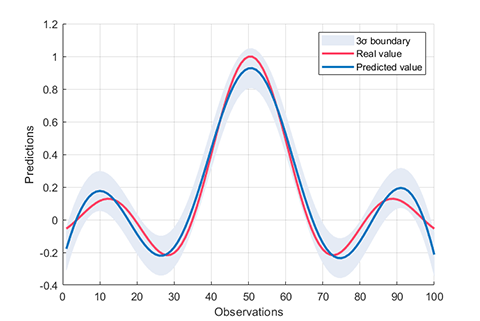2021.08.31 更新（V2.1） 源代码链接 1.  MathWorks 的 File Exchange Relevance Vector Machine (RVM) - File Exchange - MATLAB Central (mathworks.com) 2. GitHub https://github.com/iqiukp/Relevance-Vector-Machine-RVM 主要特点 二分类学习（RVC）和回归学习（RVR）支持多种核函数 (linear, gaussian, polynomial, sigmoid, laplacian)支持混合核函数（K = w1×K1+w2×K2+...+wn×Kn)支持基于贝叶斯超参数优化、遗传算法和粒子群算法的 RVM 参数优化 注意 仅支持 R2016b 以上的 MATLAB提供了多个示例文件，每个文件的开头都有对应的介绍此代码仅供参考 使用说明 01. 二分类学习 数据来源：MATLAB 自带的 fisheriris 数据集 clc clear all close all addpath(genpath(pwd)) % use fisheriris dataset load fisheriris inds = ~strcmp(species, 'setosa'); data_ = meas(inds, 3:4); label_ = species(inds); cvIndices = crossvalind('HoldOut', length(data_), 0.3); trainData = data_(cvIndices, :); trainLabel = label_(cvIndices, :); testData = data_(~cvIndices, :); testLabel = label_(~cvIndices, :); % kernel function kernel = Kernel('type', 'gaussian', 'gamma', 0.2); % parameter parameter = struct( 'display', 'on',...                     'type', 'RVC',...                     'kernelFunc', kernel); rvm = BaseRVM(parameter); % RVM model training, testing, and visualization rvm.train(trainData, trainLabel); results = rvm.test(testData, testLabel); rvm.draw(results)复制代码结果 *** RVM model (classification) train finished *** running time            = 0.1604 seconds iterations              = 20 number of samples       = 70 number of RVs           = 2 ratio of RVs            = 2.8571% accuracy                = 94.2857% *** RVM model (classification) test finished *** running time            = 0.0197 seconds number of samples       = 30 accuracy                = 96.6667%复制代码以及对应的混淆矩阵图和预测结果图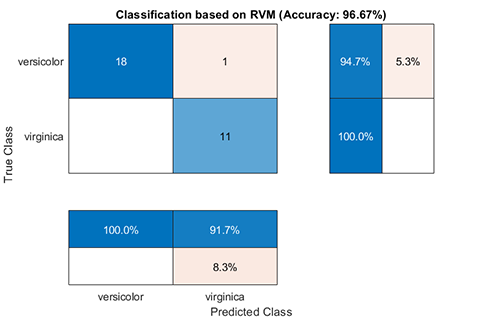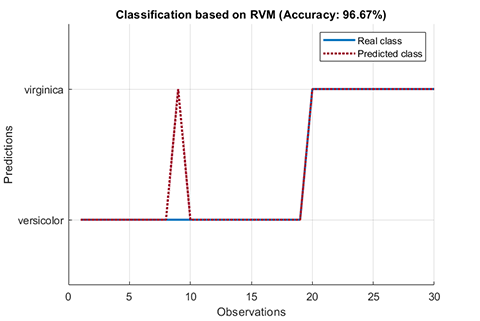02. 回归学习（RVR） 数据来源：sinc 曲线数据 clc clear all close all addpath(genpath(pwd)) % sinc funciton load sinc_data trainData = x; trainLabel = y; testData = xt; testLabel = yt; % kernel function kernel = Kernel('type', 'gaussian', 'gamma', 0.1); % parameter parameter = struct( 'display', 'on',...                     'type', 'RVR',...                     'kernelFunc', kernel); rvm = BaseRVM(parameter); % RVM model training, testing, and visualization rvm.train(trainData, trainLabel); results = rvm.test(testData, testLabel); rvm.draw(results)复制代码结果 *** RVM model (regression) train finished *** running time            = 0.1757 seconds iterations              = 76 number of samples       = 100 number of RVs           = 6 ratio of RVs            = 6.0000% RMSE                    = 0.1260 R2                      = 0.8821 MAE                     = 0.0999 *** RVM model (regression) test finished *** running time            = 0.0026 seconds number of samples       = 50 RMSE                    = 0.1424 R2                      = 0.8553 MAE                     = 0.1106复制代码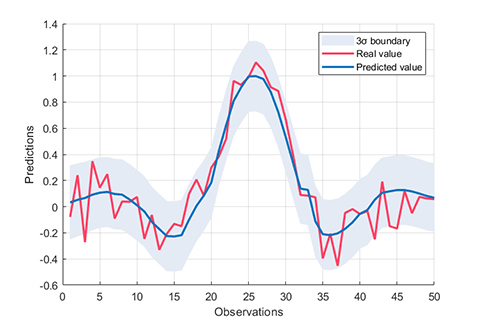03. 核函数的设置 利用 Kernel 类来设置不同类型的核函数 %{         type   -                  linear      :  k(x,y) = x'*y         polynomial  :  k(x,y) = (γ*x'*y+c)^d         gaussian    :  k(x,y) = exp(-γ*||x-y||^2)         sigmoid     :  k(x,y) = tanh(γ*x'*y+c)         laplacian   :  k(x,y) = exp(-γ*||x-y||)                 degree -  d         offset -  c         gamma  -  γ %} kernel = Kernel('type', 'gaussian', 'gamma', value); kernel = Kernel('type', 'polynomial', 'degree', value); kernel = Kernel('type', 'linear'); kernel = Kernel('type', 'sigmoid', 'gamma', value); kernel = Kernel('type', 'laplacian', 'gamma', value);复制代码例如，计算 X 和 Y 的高斯核函数矩阵： X = rand(5, 2); Y = rand(3, 2); kernel = Kernel('type', 'gaussian', 'gamma', 2); kernelMatrix = kernel.computeMatrix(X, Y); >> kernelMatrix kernelMatrix =     0.5684    0.5607    0.4007     0.4651    0.8383    0.5091     0.8392    0.7116    0.9834     0.4731    0.8816    0.8052     0.5034    0.9807    0.7274复制代码 04. 混合核函数（K = w1×K1+w2×K2+...+wn×Kn) 以高斯核函数与多项式核函数的混合为例。 clc clear all close all addpath(genpath(pwd)) % sinc funciton load sinc_data trainData = x; trainLabel = y; testData = xt; testLabel = yt; % kernel function kernel_1 = Kernel('type', 'gaussian', 'gamma', 0.3); kernel_2 = Kernel('type', 'polynomial', 'degree', 2); kernelWeight = [0.5, 0.5]; % parameter parameter = struct( 'display', 'on',...                     'type', 'RVR',...                     'kernelFunc', [kernel_1, kernel_2],...                     'kernelWeight', kernelWeight); rvm = BaseRVM(parameter); % RVM model training, testing, and visualization rvm.train(trainData, trainLabel); results = rvm.test(testData, testLabel); rvm.draw(results)复制代码 05. 单核RVM的参数优化 以贝叶斯超参优化为例，优化高斯核函数的参数。 clc clear all close all addpath(genpath(pwd)) % use fisheriris dataset load fisheriris inds = ~strcmp(species, 'setosa'); data_ = meas(inds, 3:4); label_ = species(inds); cvIndices = crossvalind('HoldOut', length(data_), 0.3); trainData = data_(cvIndices, :); trainLabel = label_(cvIndices, :); testData = data_(~cvIndices, :); testLabel = label_(~cvIndices, :); % kernel function kernel = Kernel('type', 'gaussian', 'gamma', 5); % parameter optimization opt.method = 'bayes'; % bayes, ga, pso opt.display = 'on'; opt.iteration = 20; % parameter parameter = struct( 'display', 'on',...                     'type', 'RVC',...                     'kernelFunc', kernel,...                     'optimization', opt); rvm = BaseRVM(parameter); % RVM model training, testing, and visualization rvm.train(trainData, trainLabel); results = rvm.test(trainData, trainLabel); rvm.draw(results)复制代码优化结果 *** RVM model (classification) train finished *** running time            = 13.3356 seconds iterations              = 88 number of samples       = 70 number of RVs           = 4 ratio of RVs            = 5.7143% accuracy                = 97.1429% Optimized parameter  table     gaussian_gamma     ______________         7.8261    *** RVM model (classification) test finished *** running time            = 0.0195 seconds number of samples       = 70 accuracy                = 97.1429%复制代码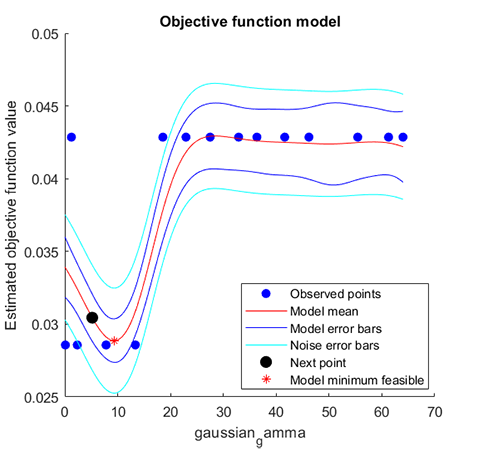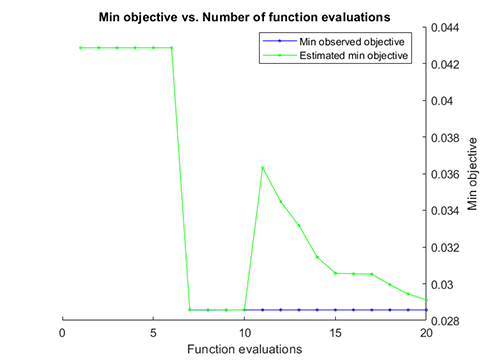06. 混合核 RVM的参数优化 优化对象为：多个核函数自身的参数，以及对应的权重，数据来源于某 UCI 数据集。 clc clear all close all addpath(genpath(pwd)) % data load UCI_data trainData = x; trainLabel = y; testData = xt; testLabel = yt; % kernel function kernel_1 = Kernel('type', 'gaussian', 'gamma', 0.5); kernel_2 = Kernel('type', 'polynomial', 'degree', 2); % parameter optimization opt.method = 'bayes'; % bayes, ga, pso opt.display = 'on'; opt.iteration = 30; % parameter parameter = struct( 'display', 'on',...                     'type', 'RVR',...                     'kernelFunc', [kernel_1, kernel_2],...                     'optimization', opt); rvm = BaseRVM(parameter); % RVM model training, testing, and visualization rvm.train(trainData, trainLabel); results = rvm.test(testData, testLabel); rvm.draw(results)复制代码结果 *** RVM model (regression) train finished *** running time            = 24.4042 seconds iterations              = 377 number of samples       = 264 number of RVs           = 22 ratio of RVs            = 8.3333% RMSE                    = 0.4864 R2                      = 0.7719 MAE                     = 0.3736 Optimized parameter  1×6 table     gaussian_gamma    polynomial_gamma    polynomial_offset    polynomial_degree    gaussian_weight    polynomial_weight     ______________    ________________    _________________    _________________    _______________    _________________         22.315             13.595               44.83                  6               0.042058             0.95794      *** RVM model (regression) test finished *** running time            = 0.0008 seconds number of samples       = 112 RMSE                    = 0.7400 R2                      = 0.6668 MAE                     = 0.4867复制代码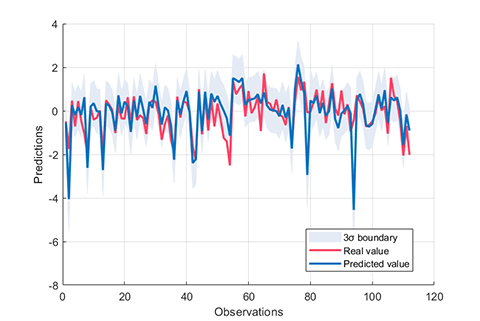07. 交叉验证 支持 K 折交叉验证和留出法交叉验证。 比如，5 折交叉验证： parameter = struct( 'display', 'on',...                             'type', 'RVC',...                             'kernelFunc', kernel,...                             'KFold', 5);复制代码比如，验证集比例为0.3的留出法交叉验证： parameter = struct( 'display', 'on',...                             'type', 'RVC',...                            'kernelFunc', kernel,...                            'HoldOut', 0.3);复制代码 08. 其它设置 %% custom optimization option %{           opt.method = 'bayes'; % bayes, ga, pso     opt.display = 'on';     opt.iteration = 20;     opt.point = 10;     % gaussian kernel function     opt.gaussian.parameterName = {'gamma'};     opt.gaussian.parameterType = {'real'};     opt.gaussian.lowerBound = 2^-6;     opt.gaussian.upperBound = 2^6;     % laplacian kernel function     opt.laplacian.parameterName = {'gamma'};     opt.laplacian.parameterType = {'real'};     opt.laplacian.lowerBound = 2^-6;     opt.laplacian.upperBound = 2^6;     % polynomial kernel function     opt.polynomial.parameterName = {'gamma'; 'offset'; 'degree'};     opt.polynomial.parameterType = {'real'; 'real'; 'integer'};     opt.polynomial.lowerBound = [2^-6; 2^-6; 1];     opt.polynomial.upperBound = [2^6; 2^6; 7];     % sigmoid kernel function     opt.sigmoid.parameterName = {'gamma'; 'offset'};     opt.sigmoid.parameterType = {'real'; 'real'};     opt.sigmoid.lowerBound = [2^-6; 2^-6];     opt.sigmoid.upperBound = [2^6; 2^6]; %} %% RVM model parameter %{     'display'    :   'on', 'off'     'type'       :   'RVR', 'RVC'     'kernelFunc' :   kernel function     'KFolds'     :   cross validation, for example, 5     'HoldOut'    :   cross validation, for example, 0.3     'freeBasis'  :   'on', 'off'     'maxIter'    :   max iteration, for example, 1000 %}复制代码luoxiao5566 发表于 2019-1-16 18:35:44
 楼主，为什么我用你的程序可以拟合数据，但是不能预测？xiaodong2019 发表于 2019-2-28 16:42:08
 非常感谢博主的分享，但是有个疑问麻烦帮忙解答： 我把sinc函数改为sin函数后，发现无法实现非线性拟合，拟合曲线偏差严重，另外相关向量曲线报错，这是什么原因？ 希望能加博主的微信，可以发到我邮箱281188347@qq.com，谢谢！

sin(x)函数无法拟合iqiukp 发表于 2019-2-28 17:57:22
 本帖最后由 iqiukp 于 2019-2-28 18:00 编辑 xiaodong2019 发表于 2019-2-28 16:42 非常感谢博主的分享，但是有个疑问麻烦帮忙解答： 我把sinc函数改为sin函数后，发现无法实现非线性拟合，拟 ... 报错的原因： 1. 训练集中的相关向量数据点标记范围超过了样本个数，导致第一幅图的第三条曲线报错（即相关向量数据点），这个问题笔者以后再修复。 2. 可以直接绕过figure1，直接看figure2的拟合效果（测试集） 拟合性能差的原因：相关向量机的重要参数为核宽度，即rvm_train.m中的sigma： % kernei width sigma = 5.5;  复制代码 提高拟合性能需要调整该参数，笔者试了试，在sigma等于3以下的时候，训练集和测试集的拟合效果为：可以看出几乎完全重合了。xiaodong2019 发表于 2019-2-28 23:30:23
 iqiukp 发表于 2019-2-28 17:57 报错的原因： 1. 训练集中的相关向量数据点标记范围超过了样本个数，导致第一幅图的第三条曲线报错（即相 ... 非常感谢博主的解答，修改核宽度后，sin(x)拟合曲线确实与函数曲线一致。     但是本人将训练函数替换为具体x,y数据后（如图1），在给定令一组x’数据后利用训练模型预测y'时得到的拟合曲线与训练曲线差别较大（如图2），且改变核宽度仍无效果，请问问题出在哪里？进一步，如果我的训练输入数据不止x一组，但只有一个y输出，请问如何开展训练和预测？     如何可以的话，希望能加上博主的扣扣或微信，本人扣扣（281188347），谢谢！iqiukp 发表于 2019-3-4 20:29:51
 xiaodong2019 发表于 2019-2-28 23:30 非常感谢博主的解答，修改核宽度后，sin(x)拟合曲线确实与函数曲线一致。     但是本人将训练函数替换为 ... 1. 你把新的训练数据通过代码的方式贴上来，我这边试试。 2. 你的意思是多个自变量，一个因变量？这种情况的处理方法也是一样的，只要保证数据的行方向是样本，列方向是特征（属性）就行。部分情况可能还得先预处理数据。xiaodong2019 发表于 2019-3-5 10:21:02
 iqiukp 发表于 2019-3-4 20:29 1. 你把新的训练数据通过代码的方式贴上来，我这边试试。 2. 你的意思是多个自变量，一个因变量？这种情 ... 多谢博主解答，该问题原因已经找到，是输入数据未归一化，而且核宽度仍然需要小于3以下。现在存在两个 疑惑： 一是整个训练模型是不是只有核宽度一个参数需要适时调整大小，还有没有其他需要参数，如何影响拟合结果；二是该语句（plot(X(model.rv_index),Y(model.rv_index),'go',  'LineWidth',1.5,'MarkerSize',8)）除了博主给出的sinc函数外一直报错（“索引超出矩阵维度”），例如换成sin函数，该语句是用来画出相关性好的训练数据的曲线么？为什么只有几个点？能否帮忙给出解决方案，谢谢！iqiukp 发表于 2019-3-5 11:33:49
 本帖最后由 iqiukp 于 2019-3-5 11:35 编辑 xiaodong2019 发表于 2019-3-5 10:21 多谢博主解答，该问题原因已经找到，是输入数据未归一化，而且核宽度仍然需要小于3以下。现在存在两个 疑 ... 1. 在SB2_Release_200工具箱里面还有一个（迭代次数）可以设置。 在rvm_train.m文件里面可以看到有个UserOptions, 工具箱默认是10000次（可以看工具箱的SB2_UserOptions.m文件） % SETTINGS = SB2_ParameterSettings('NoiseStd',0.1); OPTIONS = SB2_UserOptions('diagnosticLevel','medium','monitor',10, ...     'diagnosticFile', 'logfile.txt'); [PARAMETER, HYPERPARAMETER, DIAGNOSTIC] = ...     SparseBayes('Gaussian', BASIS, Y, OPTIONS);复制代码可以尝试把上面的OPTIONS 改为： OPTIONS = SB2_UserOptions('Iterations',10000, 'diagnosticLevel','medium', 'monitor',10, ...     'diagnosticFile', 'logfile.txt');复制代码10000是迭代次数，可以根据你的需求改成其他数字，不过RVM的默认精度已经够高了，再修改这个参数意义不是很大，重点还是核宽度那个参数。 除此之外，最基本的RVM算法应该就没有可调的参数了。你可以关注一下近年来针对RVM原理层面的改进算法，或许有可以参考的地方。 2. 你可以先看看 ‘model’ 这个变量的意思。 % SETTINGS = SB2_ParameterSettings('NoiseStd',0.1); OPTIONS = SB2_UserOptions('diagnosticLevel','medium','monitor',10, ...     'diagnosticFile', 'logfile.txt'); [PARAMETER, HYPERPARAMETER, DIAGNOSTIC] = ...     SparseBayes('Gaussian', BASIS, Y, OPTIONS); % Locate = find(PARAMETER.Relevant == L+1); % PARAMETER.Relevant(Locate)=[]; % PARAMETER.Value(Locate)=[]; model.rv_index = PARAMETER.Relevant; model.rv_mu = PARAMETER.Value; model.width = sigma; model.X = X; model.beta = HYPERPARAMETER.beta; model.sigma = DIAGNOSTIC.Sigma;复制代码可以看到，model是一个结构体，整合了RVM模型的一些参数。其中 model.rv_index 是训练集里面相关向量的下标（实际上也是训练集里面的样本）。plot(X(model.rv_index),Y(model.rv_index),'go','LineWidth',1.5,'MarkerSize',8)复制代码实际上该语句的功能就是想把训练集中那些属于“相关向量”的样本给标记出来，因此只有少数几个点，稀疏性好。另外，报错的那个原因已经找到，但目前暂时没有找到解决的方法。hayixiao 发表于 2019-3-8 14:43:12
 请教楼主： 如果用DE来优化楼主的RVM算法，只能优化核参数sigma吗？一般的论文文献中优化的是至少两个参数：α、σ。如果要优化楼主的算法，适应度函数如何构造？iqiukp 发表于 2019-5-11 15:32:42
 本帖最后由 iqiukp 于 2019-5-11 22:31 编辑 2019.5.11 更新Relevance Vector Machine (RVM).zip (1.24 MB, 下载次数: 173) 2019-5-11 15:31 上传 点击文件名下载附件 1.  修复部分错误；2.  优化了部分代码；3.  修改了原工具包的SparseBayes.m文件 示例：clc clear all close all addpath(genpath(pwd)) % sinc funciton fun = @(x) sin(abs(x))/abs(x); % training samples x = linspace(-10,10,100); y = arrayfun(fun,x); Xtrain = x'; Ytrain = y'; % testing samples xtest = linspace(-10,10,30); ytest = arrayfun(fun,xtest); Xtest = xtest'; Ytest = ytest'; % Train RVM model model = rvm_train(Xtrain,Ytrain,'sigma',5.5,'bias',1); % Test RVM model [y_mu,y_var] = rvm_test(model,Xtest); % Plot the training results plottrainingResult(Xtrain,Ytrain,model) % Plot the testing results plottestingResult(Xtest,Ytest,y_mu,y_var)复制代码 模型训练结果及测试样本预测结果iqiukp 发表于 2019-5-12 17:26:27
 hayixiao 发表于 2019-3-8 14:43 请教楼主： 如果用DE来优化楼主的RVM算法，只能优化核参数sigma吗？一般的论文文献中优化的是至少两个参数 ... 不好意思，现在才回复你的问题。 RVM的回归是把权重W的估计转化为α、σ超参数的估计，一般论文里面用的是第二类最大似然估计方法来估计超参数的最优值。程序里面的sigma调整严格来说不算参数优化范畴，只是修改了基函数矩阵的数值。 1. 要是优化核参数sigma的话，适应度函数的输入是sigma，输出是一般是反映预测精度的指标，如RMSE和R2这些，这个是通用的，无论你用哪种优化算法。 2. 要是从RVM自身的超参数优化出发的话，这方面的文献不多，顶多是优化了超参数估计的迭代过程，笔者的研究领域没怎么涉及到这方面的内容，你可以多查阅相关的文献。iqiukp 发表于 2019-5-11 15:32 2019.5.11 更新 楼主，我做的是多输入单输出的相关向量机预测模型，可以加QQ请教程序该怎么改吗，幺幺幺肆零叁柒幺肆贰，谢谢~iqiukp 发表于 2019-5-15 16:43:28
 鱼鱼_jFfBF 发表于 2019-5-15 16:26 楼主，我做的是多输入单输出的相关向量机预测模型，可以加QQ请教程序该怎么改吗，幺幺幺肆零叁柒幺肆贰， ... 把训练数据X(M*N)，Y(M*1)设定为： 1. M为样本个数，N为变量个数（即输入个数）。 2. 根据你的数据类型，可能需要归一化处理（mapminamx）或者标准化处理（zscore）。qsmmmmmm 发表于 2019-5-16 11:05:02
 楼主我想请教一下，关于实际值 ytest 和预测值 y_mu 之间的残差有没有定量描述的指标？也就是评价预测精度的指标？万分感谢如果做多输入多输出，是个否可以呢，请教qsmmmmmm 发表于 2019-5-16 11:46:50
 iqiukp 发表于 2019-5-15 16:43 把训练数据X(M*N)，Y(M*1)设定为： 1. M为样本个数，N为变量个数（即输入个数）。 2. 根据你的数据类型， ... 我按照这个，训练时输入X（44，4），Y（44，1），并归一化，得到model， 在预测时输入Xtest（44，1），Ytest（44，1） 运行时报错： 错误使用  * 用于矩阵乘法的维度不正确。请检查并确保第一个矩阵中的列数与第二个矩阵中的行数匹配。要执行按元素相乘，请使用 '.*'。 出错 computeKM (line 13) K = exp((bsxfun(@minus,bsxfun(@minus,2*x*y',sx),sy'))/sigma^2); 出错 rvm_test (line 24) BASIS = computeKM(X,model.X,model.width); 出错 prediction (line 24) [y_mu,y_var] = rvm_test(model,Xtest);%得到预测值y_muqsmmmmmm 发表于 2019-5-16 11:59:07
 qsmmmmmm 发表于 2019-5-16 11:46 我按照这个，训练时输入X（44，4），Y（44，1），并归一化，得到model， 在预测时输入Xtest（44，1），Yt ... 初步判断是model参数里面的model.X是用来训练的X，应该是预测时和训练时用的是同一个核函数，造成前后输入维度不同。。iqiukp 发表于 2019-5-16 12:11:18
 本帖最后由 iqiukp 于 2019-5-16 12:30 编辑 龙宝百 发表于 2019-5-16 11:06 如果做多输入多输出，是个否可以呢，请教 如果不考虑多个输出之间的相关性，可以用笔者提供的RVM程序单独预测每个输出；如果考虑多个输出之间的相关性，可以参考这篇多输入多输出的文章： Ha Y, Zhang H. Fast multi-output relevance vector regression[J]. Economic Modelling, 2019.Ha_Zhang_2019_Fast multi-output relevance vector regression.pdf (1.35 MB, 下载次数: 124) 2019-5-16 12:23 上传 点击文件名下载附件 文章的作者也给出了源码FMRVR.zip (11.34 KB, 下载次数: 117) 2019-5-16 12:25 上传 点击文件名下载附件 源码的地址（把地址中的中文去掉）： ht学术tps://w学术w2.math学术works.cn/matla学术bcentral/fi学术leexchange/49131-fast-multi-output-relevance-vector-r学术egression?requestedDoma学术in=zhiqiukp 发表于 2019-5-16 12:13:10
 qsmmmmmm 发表于 2019-5-16 11:46 我按照这个，训练时输入X（44，4），Y（44，1），并归一化，得到model， 在预测时输入Xtest（44，1），Yt ... Xtest的维度错误。测试样本的个数没有限制，但是特征维度要和Xtrain一致。iqiukp 发表于 2019-5-16 12:14:17
 本帖最后由 iqiukp 于 2019-5-16 16:43 编辑 qsmmmmmm 发表于 2019-5-16 11:59 初步判断是model参数里面的model.X是用来训练的X，应该是预测时和训练时用的是同一个核函数，造成前后输 ... 嗯嗯，是这个问题。iqiukp 发表于 2019-5-16 12:19:00
 qsmmmmmm 发表于 2019-5-16 11:05 楼主我想请教一下，关于实际值 ytest 和预测值 y_mu 之间的残差有没有定量描述的指标？也就是评价预测精度 ... 定量描述的指标目前常用的有3个： 1. RMSE:  均方根误差，其值越小越好。 2.  CD:    决定系数 coefficient of determination (即相关系数的平方)，其值越大越好。 3.  MAE:   平均绝对误差 mean absolute error，其值越小越好。 关于这几个指标的计算公式和使用区别网上相关资料很多，这里就不赘述了。qsmmmmmm 发表于 2019-5-16 14:03:47
 iqiukp 发表于 2019-5-16 12:19 定量描述的指标目前常用的有3个： 1. RMSE:  均方根误差，其值越小越好。 我在一篇文章里看到用贝叶斯因子来衡量，代表训练过后对残差进行的变换（高斯变换），其中用到了残差的均值与标准差，代表用模型预测时对残差的高斯变换， 最后再做如下计算，，B的大小来衡量ytest偏离ytrain的程度，进而识别数据与训练时用的基准数据的偏差，在您看来这种方法有没有指导意义？iqiukp 发表于 2019-5-16 16:37:42
 qsmmmmmm 发表于 2019-5-16 14:03 我在一篇文章里看到用贝叶斯因子来衡量，代表训练过后对残差进行的变换（高斯变换），其中用到了残差的均 ... 如果你的研究重点不是这个评价方法，完全可以使用别人提出的新评价方法，通过结合多个评价指标，包括传统的指标，如RMSE这些，以及类似贝叶斯因子这种比较新颖的评价指标，都是从侧面验证你的实验结果好坏。 如果你的研究重点是回归预测的评价，既然这个贝叶斯因子方法是已经公开发表的，肯定有指导意义，因为RVM本身的模型输出就是均值和方差。进而再思考一下，这个贝叶斯因子提出的依据和原因是啥？为什么B的偏差就能衡量预测效果？以及这个贝叶斯因子的应用对象是不是也有一定的数据要求？qsmmmmmm 发表于 2019-5-17 10:07:53
 iqiukp 发表于 2019-5-16 16:37 如果你的研究重点不是这个评价方法，完全可以使用别人提出的新评价方法，通过结合多个评价指标，包括传统 ... 好的，感谢！qsmmmmmm 发表于 2019-5-19 10:15:35
 您好，我看资料说的实际值与预测值之间的残差服从高斯分布，请问一下这个在程序里面体现了吗？ 程序里面有没有算出残差呢？还是需要自己用ytest-y_mu得到残差？
 本版积分规则 回帖后跳转到最后一页
##### 热门教程